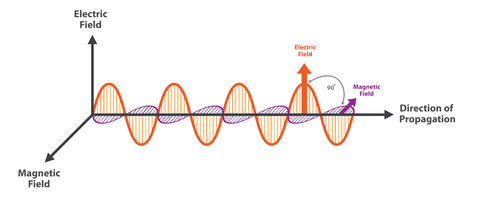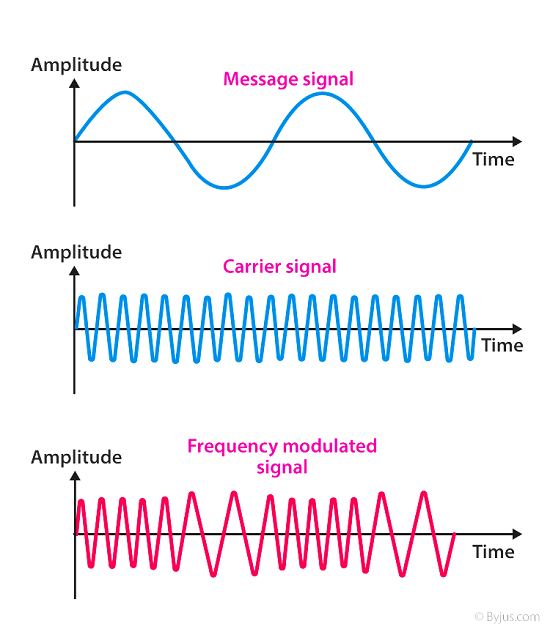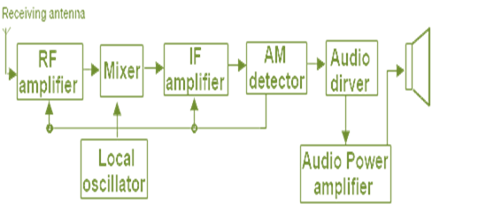# Difference Between Am and Fm

## Introduction

Wave is the disturbance in the medium to transfer energy without the transfer of mass. Waves are usually in a periodic motion like a simple harmonic oscillator. They have crests and troughs. The amplitude of the wave is the height of the wave. The distance covered between two consecutive crests is called the wavelength of the wave. The number of waves passed per second is called the frequency of the wave. Phase is not the property of waves. But it gives the relation between two signals with the same frequency.Fig:1 Propagation of wave

Classifications of the waves are transverse waves and longitudinal waves. If the particle vibrates in a perpendicular direction to that of the direction of propagation then it is called transverse wave.

Example light wave. If the particle vibrates in a parallel direction to that of the direction of propagation then it is called a longitudinal wave.

Modulation − In radio, speech and music are transmitted over longer distances. This is done by superimposing the signal onto a carrier signal of a frequency higher than the frequency of actual. This process is called modulation. The wave obtained after modulation is called a modulated wave. The wave with a high frequency on which the signal is superimposed is called a carrier wave which does not contain any information. It is denoted by,

$$\mathrm{e_{c}=E_{c}cos(\omega _{c}t+\theta )}$$

$$\mathrm{E_{c}=peak\:amplitude}$$

$\mathrm{\omega _{c}}$ = angular frequency

$\mathrm{\theta}$ = phase

Modulations are carried over by varying any one of the three parameters by taking the other two as constant. In this way, the modulation is classified into three and they are frequency modulation, amplitude modulation, and phase modulation.

## Amplitude Modulation

In amplitude modulation the amplitude of the signal is varied following the carrier signal. The frequency of the carrier waves and phase of the carrier wave does not change. No information is carried by the carrier signal but its amplitude is varied with the amplitude of the signal wave.

## Frequency Modulation

There is a change in the frequency of the carrier wave if the instantaneous signal voltage changes. It is known as frequency modulation. So amplitude and phase of the carrier wave are not changing.Frequency modulation

## What are AM, FM, and PM?

AM − It is known as amplitude modulation used to increase the amplitude of the signals.

FM − It is known as frequency modulation used to amplify the frequency of signals.

PM − Phase modulation is modulation in which the phase of the carrier wave is varied and is used for the transmission of signals.

## How does FM work?

Amplitude of the modulated wave is the same as the amplitude of the carrier wave. Its frequency changes with respect to the instantaneous signal amplitude. Whenever the signal amplitude is zero the carrier frequency is unchanged. Whenever the signal amplitude reaches a maximum on the positive side the carrier frequency also increases to maximum and it is denoted as closely spaced cycles. During the negative side maximum, the carrier frequency decreases to a minimum and it is denoted as widely spaced cycles.

## How does AM work?

It is very difficult to transmit a signal directly in the direction of propagation? Because there are some factors that affect the quantity. That is why modulation is applied. Amplitude modulation is one of the ways to modulate the signal carrying the message. The carrier signal’s amplitude is proportional to the signal carrying the message. Whenever the message signal’s amplitude is maximum in the positive direction the modulated signal’s amplitude reaches maximum. When the message signal’s amplitude is minimum in the negative direction the modulated signal’s amplitude reaches a minimum.

### Mathematical analysis

Mathematical representation of carrier wave is

$$\mathrm{e_{c}=E_{c}\:cos\omega _{c}t......(1)}$$

$$\mathrm{\omega _{c}=2\pi f_{c}}$$

$\mathrm{e_{c},E_{c}\:and\:f_{c}}$ are instantaneous voltage, amplitude, and frequency of carrier waves.

The instantaneous voltage of the modulating signal is expressed by

$$\mathrm{e_{s}=E_{s}\:cos\:\omega _{s}t.......(2)}$$

$$\mathrm{\omega _{s}=2\pi f_{s}}$$

$\mathrm{e_{c},E_{s}\:and\:f_{s}}$ are instantaneous voltage, amplitude, and frequency of signal waves.

$$\mathrm{E_{s}=mE_{c}}$$

So,

$$\mathrm{e_{s}=mE_{c}\:cos\omega _{s}t.....(3)}$$

The amplitude of amplitude modulated signal is given by

$$\mathrm{amplitude=E_{c}+ mE_{c}\:cos\:\omega _{s}t}$$

$$\mathrm{amplitude=E_{c}(1+m\:cos\:\omega _{s}t)}$$

The instantaneous voltage of amplitude modulated signal is

$$\mathrm{e=amplitude\:X\:cos\:\omega _{c}t}$$

$$\mathrm{e=E_{c}(1+m\:cos\:\omega _{c}t)cos\:\omega _{s}t}$$

$$\mathrm{e=E_{c}\:cos\:\omega_{c} t+ mE_{c}\:cos\:\omega _{s}t\:cos\:\omega _{c}t}$$

$$\mathrm{e=E_{c}\:cos\:\omega_{c} t+ mE_{c}/2(2cos\:\omega _{s}t\:cos\omega _{c}t)}$$

$$\mathrm{e=E_{c}\:cos\:\omega_{c} t+ mE_{c}/2(cos\:(\omega _{c}+\omega _{s})t+cos(\omega _{c}-\omega _{s})t}$$

$$\mathrm{since\:2cosAcosB=cos(A+B)+cos(A-B)e=E_{c}\:cos\:\omega_{c} t+ (mE_{c}/2)cos\:(\omega _{c}+\omega _{s})t+(mE_{c}/2)cos(\omega _{c}-\omega _{s})t}$$

There are three signal voltages. It includes three frequencies $\mathrm{f_{c},f_{s}+f_{s}\:and\:f_{c}+f_{s}}$

$\mathrm{f_{c}}$ the frequency of the carrier wave does not change but there are some additional frequencies $\mathrm{f_{c}+f_{s}}$ is called upper sideband frequency and $\mathrm{f_{c}-f_{s}}$ is called lower sideband frequency. They are symmetrical with respect to the carrier wave.

## Working Principle of AM Receiver in Reality

In an AM receiver an unmodulated radio frequency signal is generated by a local oscillator. This signal is mixed with the modulated R.F signal received. So the frequency of the carrier signal becomes lower with the same modulation and it is called intermediate frequency (I.F ). This intermediate signal then gets amplified and detected.

The block diagram of the AM receiver is given below. In that, the R.F amplifier receives the modulated signal from the antenna and amplifies it. And it is given to the Mixer where it is mixed with a frequency lower than from the local oscillator. And additional amplification is done by an I.F. amplifier. Then the output is given to the detector. And the detected signal is given to the A.F amplifier and then to the loudspeaker. This converts the electric signal into sound waves.## How does PM work?

Phase Modulation − The change in amplitude of the instantaneous signal makes changes in the phase of the carrier wave which is called phase modulation.

Mostly it has similarities with frequency modulation. If the modulating signal’s amplitude reaches maximum in the positive side then the carrier signal’s phase leads and shows compression. If the modulating signal’s amplitude falls and reaches a minimum on the negative side, then the carrier signal’s phase lags and shows rarefaction.

## Difference Between AM and FM

Amplitude Modulation Frequency Modulation
Variation in amplitude in accordance with carrier signal Variation of frequency in accordance with carrier signal
Frequency and phase kept constant Amplitude and phase kept constant
Poor sound quality Better sound quality
It is less complex compared to FM It is more complex.
Bandwidth is less Bandwidth is large
Power is wasted during transmission It is highly efficient as all power is utilized.

## Conclusion

Modulation is a technique used to amplify the different properties of a wave-like amplitude, frequency, bandwidth, and so forth. Different types of modulation are applied as per requirements. Every kind of modulation has some advantages as well as disadvantages. It is mostly used for telecommunication and wireless communication to transfer the data.

## FAQs

Q1. What is demodulation?

Ans. The audio signal is recovered from the modulated wave. This process is known as demodulation or detection. It is carried out in two steps. First, the negative cycle of the modulated wave is removed by rectification. And then the carrier frequency is removed by a suitable filter.

Q2. Give the application of FM modulation

Ans. Frequency modulation is applied in radar and EEG monitoring circuits.

It is mainly used in FM broadcasting. It is also applied in magnetic tape recording systems. It has a wide range of applications in satellite and microwave communication, cellular radio communication, and TV sound transmission.

Q3. What is Modulation Index?

Ans. Modulation index is the ratio of the peak voltages of the modulating signal and carrier signal.

$$\mathrm{modulation\:index=\frac{E_{s}}{E_{c}}}$$

Q4. What is the need for modulation?

Ans. The main need for the modulation is to increase the signal strength. It prevents the message from mixing with other signals.

Q5. What is the function of an antenna?

Ans. The antenna is the two-way communication used for both transmission and reception of modulated waves. During transmission, it radiates the actual sound wave into space. During the reception, it receives the modulated signal.

Updated on: 17-Jan-2023

5K+ Views